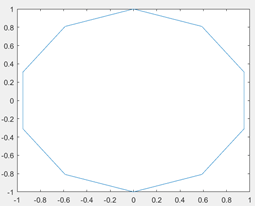# Welcome to the MATLAB Graphics MCQs Page

Dive deep into the fascinating world of MATLAB Graphics with our comprehensive set of Multiple-Choice Questions (MCQs). This page is dedicated to exploring the fundamental concepts and intricacies of MATLAB Graphics, a crucial aspect of MATLAB. In this section, you will encounter a diverse range of MCQs that cover various aspects of MATLAB Graphics, from the basic principles to advanced topics. Each question is thoughtfully crafted to challenge your knowledge and deepen your understanding of this critical subcategory within MATLAB.

Check out the MCQs below to embark on an enriching journey through MATLAB Graphics. Test your knowledge, expand your horizons, and solidify your grasp on this vital area of MATLAB.

Note: Each MCQ comes with multiple answer choices. Select the most appropriate option and test your understanding of MATLAB Graphics. You can click on an option to test your knowledge before viewing the solution for a MCQ. Happy learning!

### MATLAB Graphics MCQs | Page 1 of 7

Q1.
How can we smoothen the following graph of sin (t) and cos (t) into a circle?Answer: (a).reduce the gap between linearly spaced elements of the dependent variable t
Q2.
Which command gives a title to the graph plotted by MATLAB?
Q3.
Which command enables a title for the x-axis?
Q4.
Which command enables a title for the y-axis?
Q5.
How can several graphs for the same function be plotted on the same window?
Q6.
What is the output of the following command?
meshgrid[x,y]
Q7.
What is the output of the following code?
t=0:0.001*pi:pi/2;
plot(t,sin(t),*);
Q8.
What is the slope of the sawtooth waveform generated by the sawtooth command?